Just a place for me to keep my notes of the Introduction to Statistical Learning book.

This is by no means a comprehensive summary; just a list of points I wish to remember.

# Chapter 2: Statistical Learning

Least squares is a popular method of doing regression, but one of many methods.

The accuracy of $\hat{Y}$ as a prediction for $Y$ depends on two quantities, which we will call the reducible error and the irreducible error.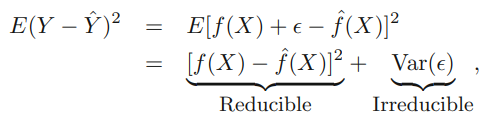We can improve the reducible error by using an more appropriate statistical technique. We can never improve the irreducible error, as it is introduced by the $\epsilon$ term in: $Y = f(x) + \epsilon$, where $Y$ is the true output.

$Y = f(X) + \epsilon$ : we assume this is the function which generated the data. It has 2 components

• $f(X) = \beta_0 + \beta_1X_1 + ... + \beta_pX_p$ which we assume is linear
• $\epsilon$ which we assume is from a mean 0 Gaussian

We estimate $f$ using $\hat{f}$. The predictions we make $\hat{Y}$ are generated from $\hat{f}$, in other words $\hat{Y} = \hat{f}$

$\hat{Y} = \hat{\beta_0} + \hat{\beta_1}X_1 + ... + \hat{\beta_p}X_p$

is only an estimate for

So, it obvisouly doesn’t take into account the error term $\epsilon$

There is no free lunch in statistics: no one method dominates all others over all possible data sets.

## Inference vs Prediction

Inference: to do with how the various predictors affect the output. For example, how does TV advertising spend affect sales, how does radio advertising affect, how does newspaper advertising, how does some combination of these affect sales?

Prediction: Predict the output, like, at a given level of advertising spends, what will the sales be?

Different models better at different things. If just interested in prediction, then a very complicated black-box model is fine. If interested in inference, simpler and more interpretable may be better.

### Accuracy vs Interpretability

Lasso less flexible, more interpretable: sets some params to 0.

Generalized additive models (GAMs) extend the linear model to allow for certain non-linear relationships. GAMs are more flexible than linear regression. They are also less interpretable than linear regression.

Fully non-linear methods such as bagging, boosting, and support vector machines bagging boosting with non-linear kernels, discussed in Chapters 8 and 9, are highly flexible approaches that are harder to interpret.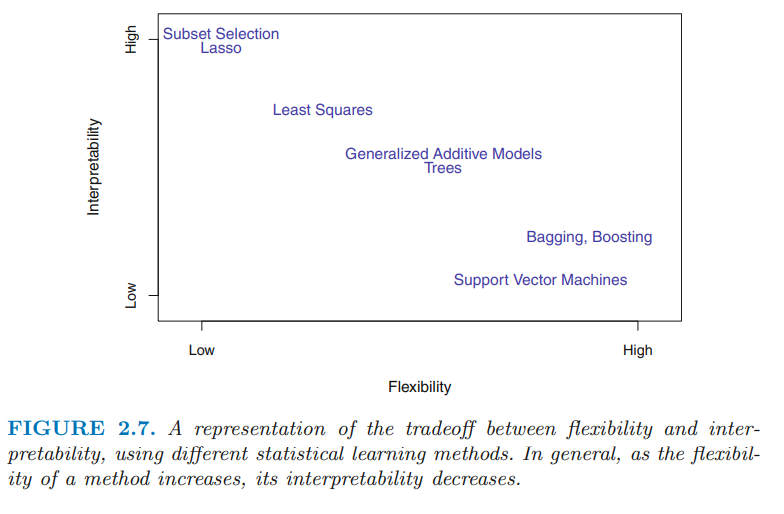## Parametric vs Non-Parametric

Statistical learning methods can be characterized as either parametric or non-parametric.

### Parametric

2 steps:

• Select a functional form (like $y = mx + c$)
• fit / train the model.

pro: assuming functional form, it may be easier (than non-parametric case) to find params. con: choosing bad functional form is… bad.

### Non-Parametric

No explict assumptions about functional form.

pro: avoid danger of choosing a bad functional form con: since non-parametric do not reduce the problem to finding a fixed number of parameters, lots more training data needed.

Thin-plate spline is an example of non-parametric. Set a “smoothness” parameter.

### Train vs Test MSE

When a given method yields a small training MSE but a large test MSE, we are said to be overfitting the data.

Cross-validation is a method for estimating test MSE using the training data.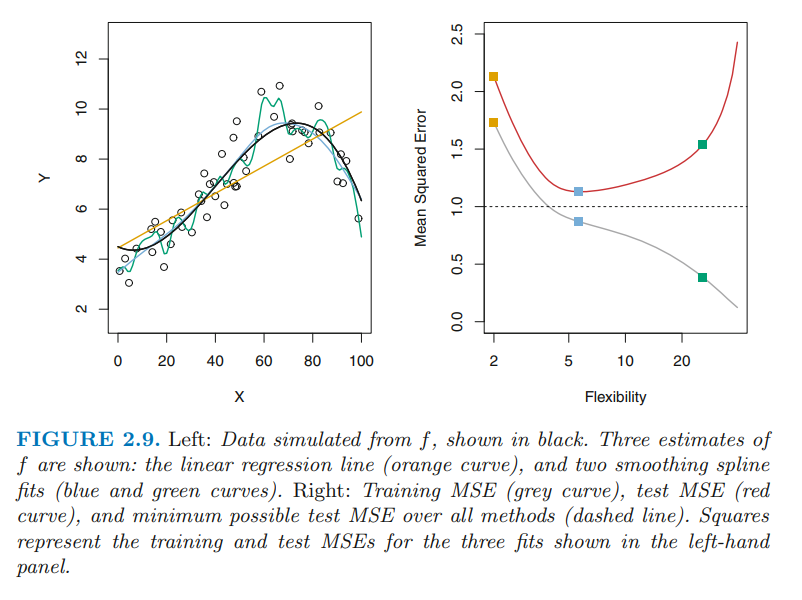Expected (since, expected over value of $\hat{f}(x_0)$ over many number of training sets used to estimate $f$) test MSE of a given $x_0$ can be broken down into:

• The variance of $\hat{f}(x_0)$
• Square bias of $\hat{f}(x_0)$
• The variance of the $\epsilon$

The last one, corresponds to irreducible error. We can control the other two somewhat by changing our choice of model.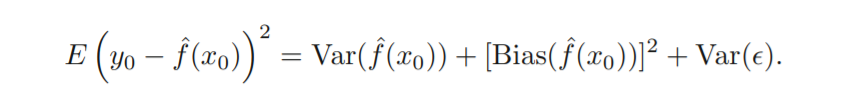In this context, variance refers to how much $\hat{f}$ would change if we used a different training set (Overfitting). Bias refers to the error we introduce by approximating a (possibly very complicated) real-life problem with a simpler mathemetical model (Underfitting).

Generally, more flexible methods result in less bias. More flexible statistical methods have higher variance.

## Bayes Classifier

Error rate is minimized, on average, by a very simple classifier that assigns each observation to the most likely class, given its predictor values. Obviously we don’t know the most likely class!

The Bayes classifier produces the lowest possible test error rate, called the Bayes error rate.

The Bayes error rate is analogous to the irreducible error, discussed earlier.

## KNN

KNN classifier identifies the K points in the training data that are closest to $x_0$. Estimates class probability as proportion of these classes in the K points.

# Chapter 3: Linear Regression

New terms in this chapter:

• Interaction Effect
• Residual
• population regression line
• least squares line
• biased / unbiased estimators
• standard error
• RSE
• confidence interval
• hypothesis test
• t-statistic
• $p$-value
• $R^2$
• Total Sum of Squares
• correlation
• $F$ statistic
• forward selection
• variable selection
• synergy / interaction effect
• interaction terms
• prediction intervals
• dummy variable
• hierarchical principle
• polynomial regression
• Residual plots
• heteroscedasticity
• studentized residuals
• high leverage
• leverage statistic

This chapter reviews ideas underlying linear regression, as well as least squares approach that is commonly used to fit this model.

## Simple Linear Regression

Simple linear regression refers to predicting $Y$ from a single predictor, $X$.

Fit line that is closest to datapoints. most common measure of closeness involved minimizing least squares criterion.

The residual is the difference between the $i$th observed response value and the $i$th response value predicted by the model: $e_i = y_i-\hat{y}_i$

The residual sum of squares: RSS $= e_1^2 + e_2^2 + ... + e_n^2$

The least squares approach chooses $\hat{\beta}_1$ and $\hat{\beta}_0$ to minimize the RSS.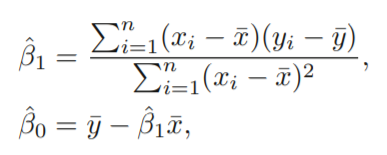The above define the least squares coefficient estimates for simple linear regression.

### Assessing the Accuracy of Coefficient Estimates

The model defined by the population regression line is the best linear approximation to the relationship between $X$ and $Y$. It is given by: $Y = \beta_0 + \beta_1 + \epsilon$.

The least squares coefficients define the least squares line.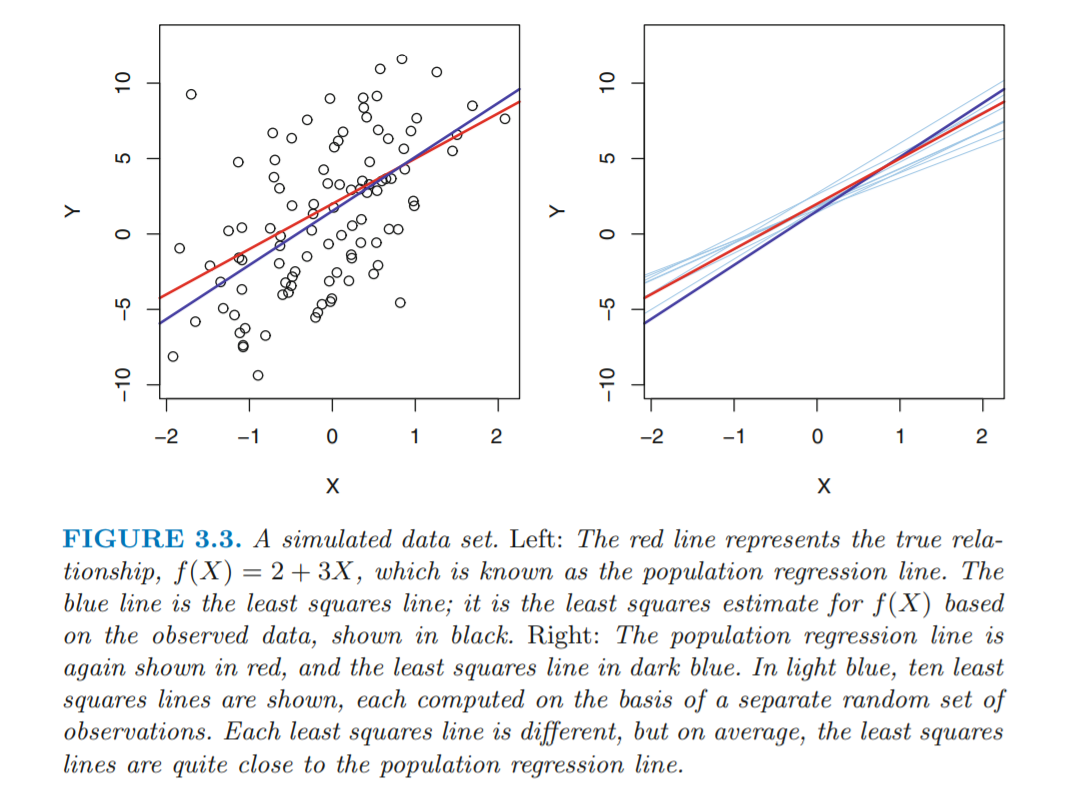#### Biased vs Unbiased Estimators

Unbiased estimator does not systematically over or under estimate the true parameter. The sample mean is an unbiased estimator: expect the sample mean to equal popln mean on average.

Similar to how we try to estimate the population mean using the sample mean, we try use the least squares coefficients to estimate the coefficients of the population least squares line. Apt analogy, as $\hat{\beta_0}$ and $\hat{\beta_1}$ are unbiased estimators, like the sample mean.

#### Standard Error

Continuing with the example of the sample mean, we’d like to be able to comment on the accuracy of the sample mean as an estimate of the population mean. That’s where standard error comes in, $SE(\hat{\mu})$.

$SE(\hat{\mu})^2$ = $Var(\hat{\mu})$ = $\frac{\sigma^2}{n}$

where $\sigma$ is the standard deviation of each of the realizations of $y_i$ (remember, you have this, as it’s in the labeled training data). So, the standard error is a ratio of 2 quantities: the bigger the standard deviation, the bigger the standard error. The more observations you have, $n$, the smaller the standard error. Makes sense, since if the data vary wildly, you need lots of observations to be sure of it. Similarly, if the data is super clustered together, then you probably just need a few obersvations. Of course, the more observations you have, the better (reflected by the denominator of the Standard Error).

The formula for the standard error holds provided that none of the observations are correlated.

We can also look at the standard errors of $\hat{\beta_0}$ and $\hat{\beta_1}$: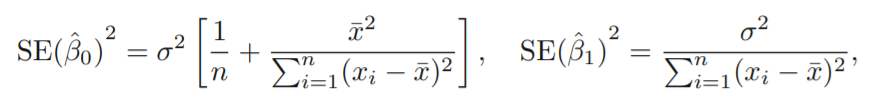Here, $\sigma^2 = Var(\epsilon)$

For these formulas to be strictly valid, we need to assume that the errors $\epsilon_i$ for each observation are uncorrelated with common variance.

Notice in the formula that $SE(\hat{\beta_1})$ is smaller when the $x_i$ are more spread out: intuitively we have more leverage to estimate a slope when this is the case.

We also see that $SE(\hat{β}_0)$ would be the same as $SE(\hat{μ})$ if $\bar{x}$ were zero (in which case $\hat{β}_0$ would be equal to $\bar{y}$).

In the above formulae, we needed $\sigma^2$ which was equal to $Var(\epsilon)$. In general, this quantity is not known, but it can be estimated from the data. The estimate for this is the residual standard error

$RSE = \sqrt{RSS / (n - 2)}$

Standard errors can be used to compute confidence intervals - so, you can say with 95% confidence that $\hat{β}_0$ and $\hat{β}_1$ lie within a certain range of values.

Standard errors can also be used to perform hypothesis tests. The most common hypothesis test involves checking whether there is a relationship between $X$ and $Y$. Mathematically, corresponding to:

$H_0 : \beta_1 = 0$

$H_1 : \beta_1 \neq 0$

Note, the hypothesis test is about $\beta_1$, but we’ll have to use $\hat{\beta_1}$. To test this hypothesis, we need to test whether $\hat{\beta}_1$ is far away enough from zero, to conclude that $\beta_1$ is non-zero. But how far away is far enough? This depends on the accuracy of $\hat{\beta}_1$, of course. And we were able to comment about its accuracy using $SE(\hat{\beta}_1)$. If we are confident in our estimate of $\hat{\beta}_1$, in other words, $SE(\hat{\beta}_1)$ is small, then even relatively small values of $\hat{\beta}_1$ can lead us to conclude that there is a relationship between $X$ and $Y$. In contrast, if we’re not that confident in the accuracy of $\hat{\beta}_1$, ie $SE(\hat{\beta}_1)$ is large, then we’ll need some pretty large values of $\hat{\beta}_1$ to convince us that there is a relationship between $X$ and $Y$.

In practice, we use t-statistic.

$t = \frac{\hat{\beta_1} - 0}{SE(\hat{\beta_1})}$

which measures the number of standard deviations away that $\hat{\beta_1}$ is from $0$. Look at it like this - the t-statistic is the ratio of the distance of the $\hat{\beta_1}$ parameter to its hypothesized value ($0$ in this case), to its standard error. The value of the t-statistic will lie somewhere on the t-distribution (with $n-2$ degrees of freedom). We want to compute the probability of observing this $t$ value, under the assumption of $\beta_1 = 0$. What is probability of having observed this value of $t$? We call this the p-value. Small p-value means it’s super unlikely to have observed it, so we reject $H_0$

### Assessing the Accuracy of The Model

Once we have rejected $H_0$ above, we need to comment on the accuracy of our model.

The quality of a linear regression fit is typically assessed using two related quantities: the residual standard error (RSE) and the $R^2$ statistic.

#### RSE

Recall, we’ve already met the $RSE = \sqrt{RSS / (n - 2)}$. We used it to estimate the value of the standard deviation of the residuals (which were Gaussian, mean 0, s.d. = ?).

In the advertising example, RSE was 3.26 (measured in thousands of units). So any prediction could be off by about 3260 units (if it’s within 1 standard deviation away from the line).

RSE is considered a measure of the lack of fit of the model to the data - is the choice of model, namely $Y = \beta_0 + \beta_1 + \epsilon$ a good one?

#### $R^2$

RSE is measured in units of Y, $R^2$ takes the form of a proportion. $R^2$ is the proportion of the variance explained by the model.

$R^2 = \frac{TSS - RSS}{TSS} = 1 - \frac{RSS}{TSS}$

$0 \leq R^2 \leq 1$

TSS measures the total variance in the response $Y$:

$TSS = \sum(y_i - \bar{y})^2$

So, it’s the variance in the actual, observed data.

RSS measures the variability that is left unexplained after performing the regression.

$RSS = \sum_{i = 1}^{n}(y_i - \hat{y}_i)^2$

So, it’s the variance left over from the difference between the actual $y_i$ and $\hat{y}_i$.

Ergo, $TSS - RSS$ measures the amount of variability in the response that is explained by the regression. $R^2$ measures the proportion of variability in in Y that can be explained using X.

In Table 3.2, the $R^2$ was 0.61, and so just under two-thirds of the variability in sales is explained by a linear regression on TV.

$R^2$ is a measure of the linear relationship between $X$ and $Y$. Correlation is also a measure of the linear relationship between $X$ and $Y$. In simple linear regression, $r^2 = R^2$. In mutliple linear regression, though, $R^2$ is different: The concept of correlation is between 2 variables, namely $X$ and $Y$ in this case. When we have $X_1, X_2, ... X_n$, the concept of correlation does not extend to these predictors and $Y$, as its no longer between 2 variables (it’s between $n + 1$). But $R^2$ still plays this same role in multiple correlation setting.

### Additional Info on lm() from R:

https://stats.stackexchange.com/questions/5135/interpretation-of-rs-lm-output

## Multiple Linear Regression

More than one predictor.

$Y = \beta_0 + \beta_1x_1 + \beta_2x2 + ... + \beta_px_p + \epsilon$

### Estimating Regression Coefficients

Can use least squares (like in simple linear regression, but requires matrix algebra).

NB: simple and multiple regression coefficients can be quite different: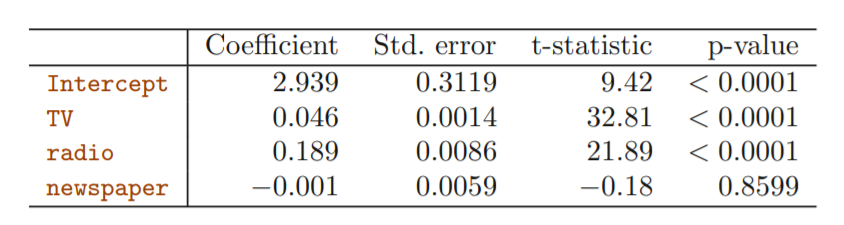Multiple Regression Coefficients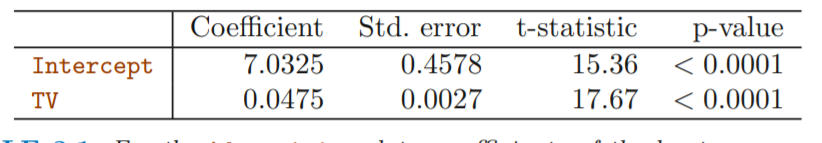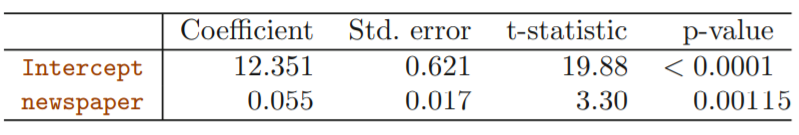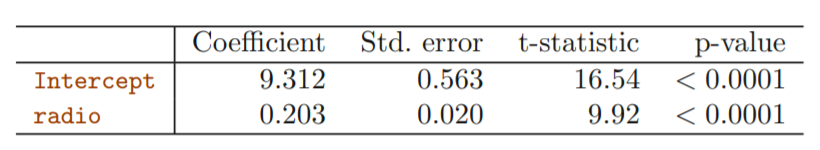Look at coefficient of Newspaper in multiple (-0.001) and simple (0.055) case. Difference stems from the fact that in the simple linear regression case, the slope term represents the average effect of a \$1000 increase in newspaper advertising, ignoring other predictors. In multiple regression setting, the coefficient for newspaper represents the average effect of increasing newspaper spending by \$1000 while holding TV and radio fixed.

How can make sense for multiple regression to suggest no relationship between sales and newspaper, where in simple regression case it does?

Look at the correlation matrix: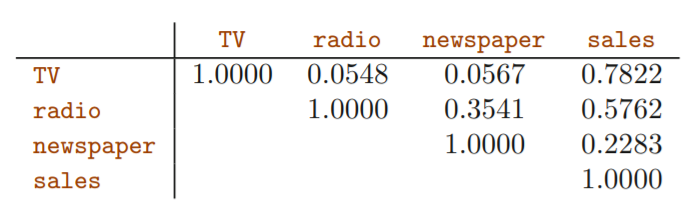Notice that the correlation between radio and newspaper is 0.35. Reveals tendency to spend more on newspaper in markets where more is spent on radio advertising.

If multiple regression is correct, then newspaper has no direct impact on sales, but radio does. In markets where more spent on radio, sales will be higher AND, as correlation matrix shows, newspaper spend will also be higher. Therefore, in simple linear regression, only looking at sales vs newspaper, we observe that higher newspaper spend tends to be associated with higher sales. Even though it doesn’t actually affect sales!!! Newspaper “takes the credit” in the absence of radio on sales.

### Is there a relationship Between The Response and Predictors?

F-statistic

The hypothesis being tested here is:

$H_0 : \beta_1 = \beta_2 = ... = \beta_p = 0$ $H_a :$ at least one $\beta_j$ is non-zero

So, we check whether or not all coefficients are zero.

Test using the F-statistic.

When there is no relationship, we expect the F-stat to take a value close to 1. If $H_a$ is true, expect it to be larger than 1. How much larger? Depends on values of $n$ and $p$ -> $n$ large, (lots of data), then needs to be just a little larger. If $n$ small, (not lots of data), $F$ needs to be bigger.

Each predictor gets its own t-statistic and p-value as well, so why also look at F-stat? Suppose $p = 100$, then there will be 100 t-statistics, one for each coefficient. In this situation, 5% (so, 5 of them), will fall below the 0.05 threshold by chance. However, the F-statistic does not suffer from this problem because it adjusts for the number of predictors.

If $p > n$ can’t use least squares.

### Deciding on Important Variables

We could look at the individual p-values of the t-statistics, but as discussed, if p is large we are likely to make some false discoveries.

How choose best model? These statistics can be used to judge quality of the model:

• Mallow’s $C_p$
• Akaike Information criterion (AIC),
• Bayesian information criterion (BIC)
• adjusted $R^2$

If got p vars, can fit $2^p$ models, so will have to get these statistcs for $2^p$ which is a shitload

variable selection -

• Forward Selection
• Backward Selection
• Mixed Selection

### Model Fit

Two of the most common numerical measures of model fit are the RSE and $R^2$, the fraction of variance explained. These quantities are computed and interpreted in the same fashion as for simple linear regression.

Recall that in simple regression, $R^2$ is the square of the correlation of the response and the variable: $Cor(X, Y)^2$. In multiple linear regression, it turns out that it equals $Cor(Y, \hat{Y} )^2$, the square of the correlation between the response and the fitted linear model.

Plotting the data: overestimates when only TV or radio is spent on, under-estimates when budget split between the two media. This non-linear relationship cannot be modeled accurately using linear regression. –> synergy / interaction effect.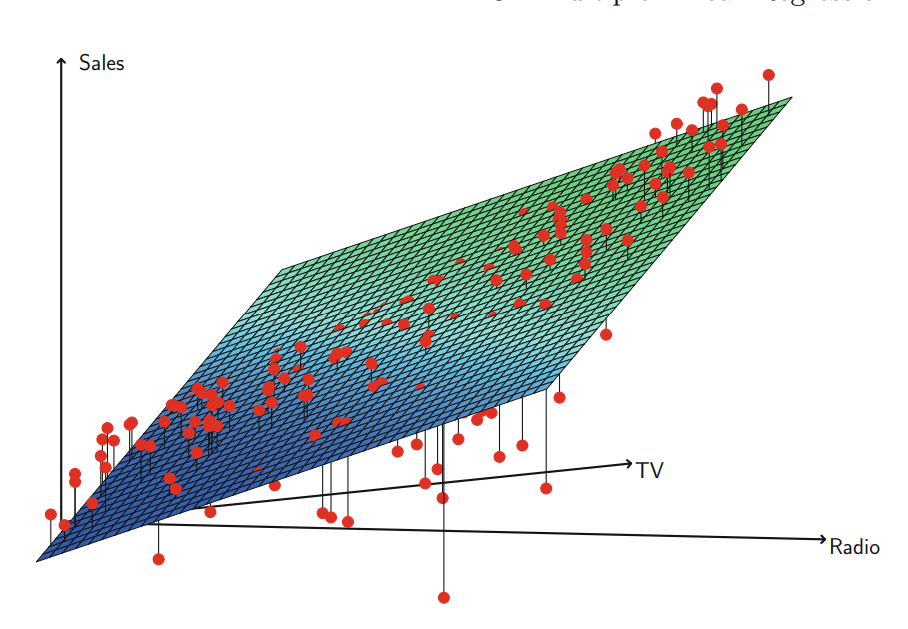### Predictions

We can compute a confidence interval in order to determine how close $\hat{Y}$ will be to $f(X)$.

Prediction intervals to see how much $\hat{Y}$ will vary from $Y$. Prediction intervals are always wider than confidence intervals because they incorporate both reducible and irreducible error. Confidence intervals only look at reducible error.

Irreducible error arises from the fact that X doesn’t completely determine $Y$ (the error term).

$\hat{Y} = \hat{\beta_0} + \hat{\beta_1}X_1 + ... + \hat{\beta_p}X_p$

is only an estimate for

$f(X) = \beta_0 + \beta_1X_1 + ... + \beta_pX_p$

So, it obvisouly doesn’t take into account the error term $\epsilon$

$Y = f(X) + \epsilon$

# Chapter 4: Classification

New Terms:

• logistic function
• maximum likelihood
• odds
• log odds / logit
• Confounding
• prior
• discriminant function

## Why not Linear Regression?

Choice of encoding affects model.

For example, suppose you’re trying to predict $Y$: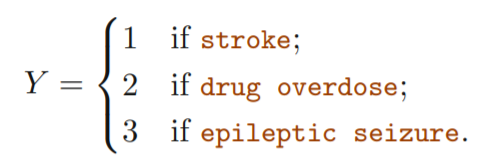This coding implies an ordering of the outcomes (in linear regression setting).

If you had to code this differently, the different coding would create a different model. So… Don’t use Linear Regression!

2 variable case is better: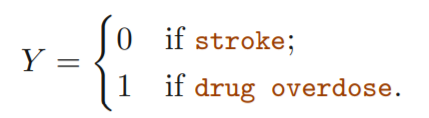But, could get estimates outside the $[0,1]$ range making them hard to interpret as probabilities.

## Logistic Regression### The Logistic Model

To avoid this problem, we must model p(X) using a function that gives outputs between 0 and 1 for all values of X. Many functions meet this description. We use the logistic function in this model:

$p(X) = \frac{e^{\beta_0 + \beta_1X}}{1 + e^{\beta_0 + \beta_1X}}$

To fit the model, use Maximum Likelihood.

Can manipulate the above equation to get:

$\frac{p(X)}{1 - p(X)} = e^{\beta_0 + \beta_1X}$

$p(X)/[1−p(X)]$ is called the odds, and can take on any value odds between $0$ and $\infty$.

Take the logarithm of both sides to get the log-odds:

$\log(\frac{p(X)}{1 - p(X)}) = \beta_0 + \beta_1X$

Let hand side is called the log-odds or logit.

Recall from Chapter 3 that in a linear regression model, $\beta_1$ gives the average change in $Y$ associated with a one-unit increase in $X$. In contrast, in a logistic regression model, increasing $X$ by one unit changes the log odds by $\beta_1$.

### Estimating the Regression Coefficients

In Chapter 3, we used the least squares approach to estimate the unknown linear regression coefficients. Although we could use (non-linear) least squares to fit the model, the more general method of maximum likelihood is preferred, since it has better statistical properties.

We seek estimates of $\beta_0$ and $\beta_1$ such that the predicted probability $\hat{p}(x_i)$ corresponds as closely as possible to the observed value (for each $i$). Mathematically, we wish to maximize the likelihood function.

The idea: Maximize the probability of seeing the data, by tuning the coefficients.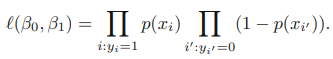The estimates $\hat{\beta}_0$ and $\hat{\beta}_1$ are chosen to maximize the above likelihood function.

In the linear regression setting, the least squares approach is in fact a special case of maximum likelihood.

Let’s look at a table of coefficients in this setting (we try to predict a default based on balance of an individual):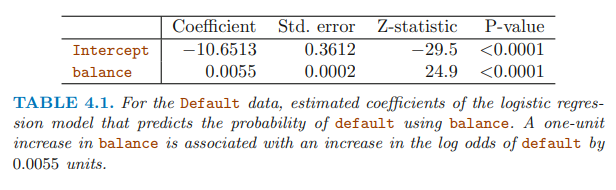Notice how the log odds are affected by the coefficient, not the direct probability.

Note that we use a Z-statistic, not a t-statistic. - In logistic and poisson regression but not in regression with gaussian errors, we know the expected variance and don’t have to estimate it separately. (https://stats.stackexchange.com/questions/60074/wald-test-for-logistic-regression)

### Making Predictions

Just plug in the values… for example, a balance of \$1000: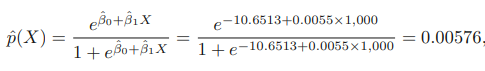Can use indicator variables, like with linear regression. For example, say we wish to predict a default based on whether or not a persion is a student: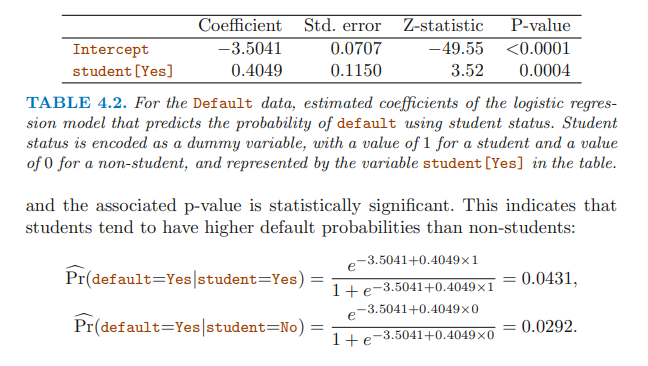### Multiple Logistic Regression

Using multiple predictors: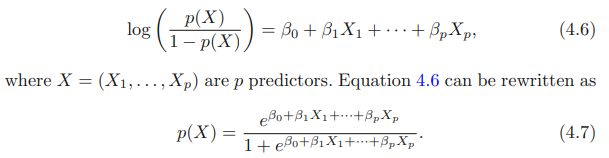So, we now try predict default based on whether or not a person is a student, and their balance.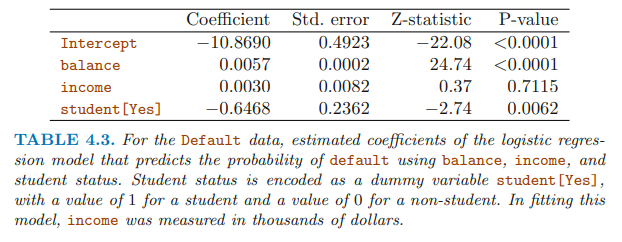Previously, the coefficient of student was positive (when it was the sole predictor). Now, it is negative. How can this be?

Look at this: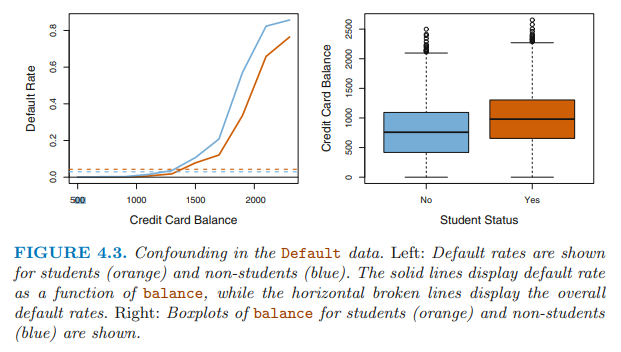Basically what the left hand graph is showing, is that for a given level of balance, a student is less risky. But there are more students with higher balances (the right hand side shows this). So, in the absence of balance data, the indicator variable “student” acts as a sort of proxy for it. In general this is known as confounding.

### Logistic for >2 Response Classes

There are extensions to the 2 class classification for logistic regression (using softmax), but this book discusses Linear Discriminant Analyis instead.

## Linear Discriminant Analysis

In logisitc regression we model $P(y = k | X = x)$, for the 2 class case. IE, we model the conditional distribution of $Y$, given the predictor(s), $X$.

In LDA, model the distribution of the predictors $X$ in each response class, and then use Bayes’ theorem to flip these into: $P(y = k | X = x)$. It’s kind of like saying: model the distribution of the predictors in each response class, and then pick the response class where it’s most likely that the given instance of $X$ came from.

When the classes are well-separated, the parameter estimates for the logistic regression model are surprisingly unstable. Linear discriminant analysis does not suffer from this problem.

If n is small and the distribution of the predictors X is approximately normal in each of the classes, the linear discriminant model is again more stable than the logistic regression model.